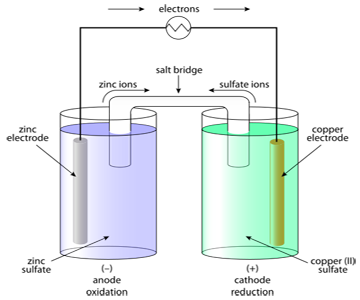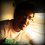# Physical Chemistry - Electrochemistry - 3Physical Chemistry - Electrochemistry - 1

## Physical Chemistry - Electrochemistry - 2

ELECTROCHEMICAL SERIESalt text

## It is series where elements are arranged in increasing order of Standard Reduction potential (or decreasing order of Standard Oxidation potential) as compared to that of S.H.E. It is experimentally determined.

Some General Characteristics of this series are:
$\bullet$ Elements near the top of the series are highly electropositive. They lose electrons readily to form cations.
$\bullet$ Elements near the bottom of the series are highly electronegative. They gain electrons readily to form anions.

## $\bullet$ Note that, the Standard Reduction Potential of Hydrogen is zero.

Keeping these points in mind, we can draw some general conclusions, such as,
$\bullet$ If metal A is situated above metal B in the electrochemical series, then A can displace B from its salt. The reason for this is that, since $E_{red}^o (A) < E_{red}^o (B)$, implying that $E_{ox}^o (A) > E_{ox}^o (B)$, thus suggesting that A has more tendency to form cations than B.

## $\bullet$ Metals situated above Hydrogen, are easily rusted. This is because these metals will have a higher tendency to oxidize (tendency to form cations). And since the definition of rusting is oxidation of metal, these metals will rust easily.

Before we can learn how to use this series to calculate the EMF of cells, we need to understand how to represent a cell as an equation. We cannot write long sentences to represent a cell everytime. It is time-consuming, and also unnecessary.

NOTATION FOR REPRESENTING A GALVANIC CELL
First of all, a Galvanic cell works through a redox reaction. One metal is oxidized, while another metal's cation is reduced. I think it will be best to approach this idea through an example.

For eg, consider the cell which uses the following reaction :
$Zn(s) + Cu^{+2}(aq) \to Zn^{+2}(aq) + Cu(s)$

$\bullet$ The interfaces, across which a P.D exists, are shown by a vertical line ( | ). In this case, the two half cells are represented as,
$Zn|Zn^{+2}$ and $Cu^{+2}|Cu$
These indicate that a P.D. exists at the $Zn$ and $Zn^{+2}$ ions interface, and similarly at the $Cu$ and $Cu^{+2}$ interface.

$\bullet$ Sometimes, a comma is observed in the formation of the half cells. For example,
$Zn,Zn(Cl)_2|Cl^{-}$
These indicate that $Zn$ and $Zn(Cl)_2$ together constitute the electrode.

$\bullet$ The contact between two solutions by means of a Salt Bridge (will be explained later) is indicated by double vertical line (||) between them. For example,
$Cu^{+2}||Zn^{+2}$

$\bullet$ The anode half-cell (oxidation half-cell) is always written on the left hand side, and the cathode half-cell (reduction half-cell) on the right, with the respective metal electrodes on the outside extremes. For example,
$Zn|Zn^{+2}||Cu^{+2}|Cu$

$\bullet$ The concentration of solution, pressure of gases and physical state of solids and liquids involved are indicated in the cell formation. For example,

## $Pt,H_2(0.9atm)|H^{+}(0.1M)||Cu^{+2}(0.1M)|Cu$

In the next post, we shall learn how to calculate the standard EMF of any cell, with the help of our new tool : Electrochemical Series.Note by Anish Puthuraya
6 years ago

This discussion board is a place to discuss our Daily Challenges and the math and science related to those challenges. Explanations are more than just a solution — they should explain the steps and thinking strategies that you used to obtain the solution. Comments should further the discussion of math and science.

When posting on Brilliant:

• Use the emojis to react to an explanation, whether you're congratulating a job well done , or just really confused .
• Ask specific questions about the challenge or the steps in somebody's explanation. Well-posed questions can add a lot to the discussion, but posting "I don't understand!" doesn't help anyone.
• Try to contribute something new to the discussion, whether it is an extension, generalization or other idea related to the challenge.

MarkdownAppears as
*italics* or _italics_ italics
**bold** or __bold__ bold
- bulleted- list
• bulleted
• list
1. numbered2. list
1. numbered
2. list
Note: you must add a full line of space before and after lists for them to show up correctly
paragraph 1paragraph 2

paragraph 1

paragraph 2

[example link](https://brilliant.org)example link
> This is a quote
This is a quote
    # I indented these lines
# 4 spaces, and now they show
# up as a code block.

print "hello world"
# I indented these lines
# 4 spaces, and now they show
# up as a code block.

print "hello world"
MathAppears as
Remember to wrap math in $$ ... $$ or $ ... $ to ensure proper formatting.
2 \times 3 $2 \times 3$
2^{34} $2^{34}$
a_{i-1} $a_{i-1}$
\frac{2}{3} $\frac{2}{3}$
\sqrt{2} $\sqrt{2}$
\sum_{i=1}^3 $\sum_{i=1}^3$
\sin \theta $\sin \theta$
\boxed{123} $\boxed{123}$

Sort by:

Nice work there

- 3 years, 9 months ago

a redox reaction is carried out at 400k. If the same reaction is carried out in presence of a catalyst at the same temperature the rate of reaction doubles . To what extent is the energy barrier loweredby the catalyst . [Use R=2cal/mol-k and log2=0.301] (a)693cal (b)554cal (c)455cal (d)231cal

- 5 years, 1 month ago

Well written. Great post!

Completely agree with Anish. Can't thank Brilliant enough!!

- 6 years ago

It will take a while to post the next note, since I have my 12th grade's Final Board examinations coming up, which will end at $14^{th}$ March, $2014$.
I will post my next note, a few days after this date. Sorry guys.

- 6 years ago

Good luck on your Boards Anish! Let us know if there is anything people on Brilliant could do to help you prepare.

Staff - 6 years ago

I have already learned too much here at Brilliant. This site is like a god gift to a student who is preparing for an entrance exam. Thank you so much (I cant tell how much) for your help..Its been a wonderful journey so far.!

- 6 years ago

Anish, Best of Luck for your boards. I also have my 10th grades finals coming up on March 3 and they end on March 26.

- 6 years ago

Good luck to you as well, do it nicely.
btw its Anish, not Danish..lol

- 6 years ago

Sorry Anish that was not intentional and thanks a lot.

- 6 years ago

Its ok Soham.

- 6 years ago

Ha ha ha ha, that's funny.

- 6 years ago